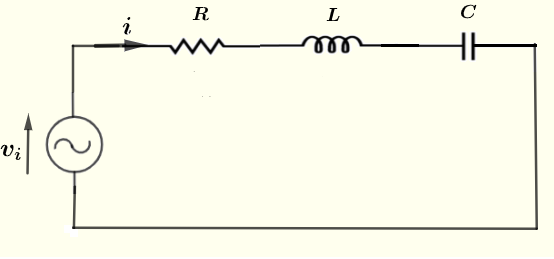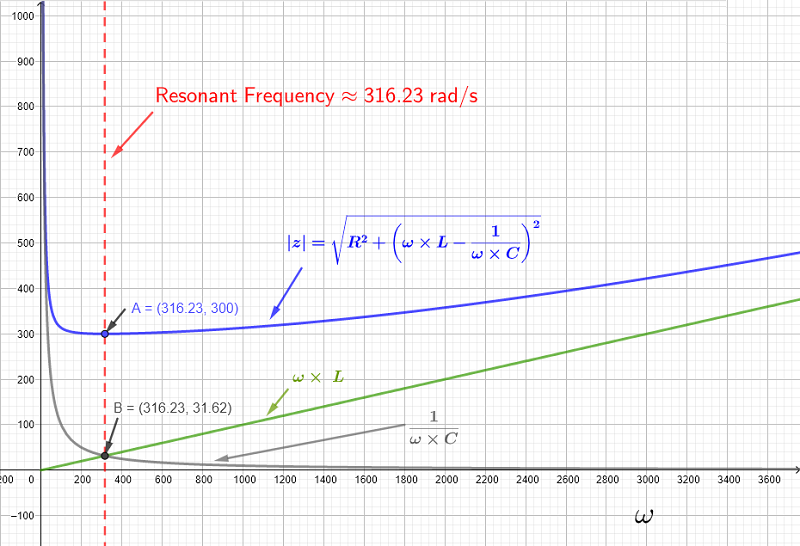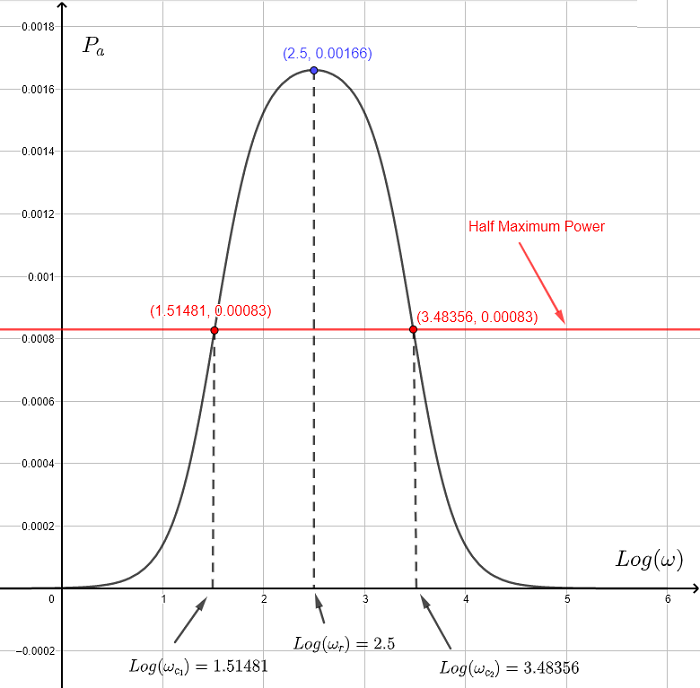# Resonant Series RLC Circuit

   

Resonant RLC series circuits and formulas of the resonant frequency , the cutoff frequencies are developed, the bandwidth and the quality factor are defined and all are used in examples with detailed solutions.
In what follows, the upper case letter $I$ is the complex (polar) form of the real current $i$ and the upper case letter $V_i$ is the complex (polar) form of the real voltage $v_i$.
A resonant series RLC circuit calculator may be used to verify the calculations of the examples below and also for more practice and investigations of these circuits.

## A - Resonant series RLC circuit

Consider the series RLC circuit shown below.For a circuit supplied by a voltage source of frequency $f$, the total impedance $Z$ of the series RLC circuitis given by:
$Z = R + j \left(\omega L - \dfrac{1}{\omega C} \right)$
The relationship between the current $I$ and the voltage $V_i$ is given by
$I = \dfrac{V_i}{Z}$
where $V_i$ and $I$ are the complex form of the voltage $v_i$ and the current $i$ respectively.
Using the definition of the magnitude of a complex number, the magnitude $|Z|$ is given by
$|Z| = \sqrt {R^2 + \left(\omega L - \dfrac{1}{\omega C} \right)^2}$
If $V_0$ is the peak value of the voltage source $v_i = V_0 \cos (\omega t)$, then the peak value $I_0$ of $I$ is given by
$I_0 = \dfrac{V_0}{ |Z| } = \dfrac{V_0}{ \sqrt {R^2 + \left(\omega L - \dfrac{1}{\omega C} \right)^2} }$
The frequency of resonance is defined as the frequency for which $I_0$ is maximum or when the magnitude of $Z$ is minimum.
Since the resistance $R$ is independent of the frequency, the minimum value of $|Z|$ occurs at $\omega = \omega_r$ such that
$\left(\omega_r L - \dfrac{1}{\omega_r C} \right) = 0$
Solve the above for $\omega_r$ to obtain the resonant frequency
$\omega_r = \dfrac{1}{\sqrt {L C}} \quad \quad (I)$
At the resonant frequency $\omega = \omega_r$, we have:
1) $Z = R$
For $V_0$, the peak value of the voltage source $v_i$, the peak value $I_0$ of $I$ is given by
2) $I_0 = \dfrac{V_0}{R}$
Let $X_L = \omega L$ and $X_C = \dfrac{1}{\omega C}$
3) $X_L = X_C$

Example 1
Let $R=300 \; \Omega$, $L = 100 \; mH$ and $C = 100 \mu F$ in the series RLC circuit above.
a) Find the resonant frequency $\omega_r$
b) Graph $|Z|$, $X_L = \omega L$, $X_C = \dfrac{1}{\omega C}$ and $I_0$ as a function of the frequency $\omega$ and discuss the graphs obtained.

Solution to Example 1
a)
The resonant frequency $\omega_r$ is given by
$\omega_r = \dfrac{1}{\sqrt {L C}} = \dfrac{1}{\sqrt{100\times10^{-3} \times 100 \times 10^{-6}}} \approx 316.23$
b)
Below are shown the graphs of $|Z|$, $X_L$ and $X_C$.
From the graphs, $|Z|$ has a minimum value equal to $R = 300 \; \Omega$ (point A)
The graphs of $X_L$ and $X_C$ intersect (point B) and are therefore $X_L = X_C$ or $\left(\omega_r L - \dfrac{1}{\omega_r C} \right) = 0$ .In the graph below is shown the current $I_0$ and is maximum at the resonant frequency $\omega_r \approx 316.23$ (after rounding to 2 decimal places)## B - Average Power in a Resonant Circuit

The average power $P_a$ delivered to the series RLC circuit is given by: $\displaystyle \quad \quad P_a = \dfrac{V_0^2}{2 |Z|} \cos \theta \quad \quad (II)$ where $\theta$ is the argument of the impedance $Z = R + j \left(\omega L - \dfrac{1}{\omega C} \right)$ and is given by
$\theta = \arctan \left( \dfrac{ \omega L - \dfrac{1}{\omega C} }{R} \right)$
Using inverse trigonometric functions properties, we have
$\tan \theta = \left( \dfrac{ \omega L - \dfrac{1}{\omega C} }{R} \right)$
$\theta$ may be assumed to be an acute angle of a right triangle as shown below. (Use the definition of the tangent of an angle in a right triangle and see that you can get $\tan \theta$ as defined above.We now use the same triangle and calculate the power factor $\cos \theta$
The hypotenuse of the triangle is calculated as follows
$AC = \sqrt {R^2 + \left( \omega L - \dfrac{1}{\omega C} \right)^2}$
$\cos \theta = \dfrac{AB}{AC} = \dfrac{R}{\sqrt {R^2 + \left( \omega L - \dfrac{1}{\omega C} \right)^2}}$
Substitute $\cos \theta$ and $|Z|$ in the formula (II) given above and express the power $P_a$ as
$\displaystyle \quad \quad P_a = \dfrac{V_0^2}{2 \sqrt {R^2 + \left(\omega L - \dfrac{1}{\omega C} \right)^2} }\dfrac{R}{\sqrt {R^2 + \left( \omega L - \dfrac{1}{\omega C} \right)^2}}$
Simplify
$\displaystyle \quad \quad P_a = \dfrac{V_0^2 R}{2 \left({R^2 + \left(\omega L - \dfrac{1}{\omega C} \right)^2} \right) } \quad \quad (III)$
At the resonant frequency $\omega_r = \dfrac{1}{\sqrt {LC}}$, we have $\left(\omega_r L - \dfrac{1}{\omega_r C} \right) = 0$ and therefore the power is maximum and equal to $P_{a max} = \dfrac{V_0^2}{2 \; R} \quad \quad (IV)$

## C - Cutoff Frequencies of a Resonant Circuit and The Quality Factor

We now define the cutoff frequencies as the frequencies $\omega_c$ at which the power $P_a(\omega)$ in (III) is half the maximum power $P_{a max}$ in (IV).
Hence we need to solve the equation
$P_a (\omega_c ) = \dfrac{1}{2} \left(\dfrac{V_0^2}{2 \; R} \right)$

$\dfrac{V_0^2 R}{2 \left({R^2 + \left(\omega_c L - \dfrac{1}{\omega_c C} \right)^2} \right) } = \dfrac{1}{2} \dfrac{V_0^2}{2 \; R}$
Simplify to
$\dfrac{ R}{2 \left({R^2 + \left(\omega_c L - \dfrac{1}{\omega_c C} \right)^2} \right) } = \dfrac{1}{4 R}$
Cross multiply, simply and rewrite the above equation as
$(\omega_c L - \dfrac {1}{\omega_c C } ) = R^2$
Solve by extracting the square root to obtain two equations
$\omega_c L - \dfrac {1}{\omega_c C} = \pm R$
Multiply all terms by $\omega_c C$ and simplify
$\omega_c^2 L C - 1 = \pm \omega_c R C$
Rewrite as quadratic equations in standard forms
$\omega_c^2 L C \pm \omega_c R C - 1 = 0$
Solve the first quadratic equation $\quad \omega_c^2 L C + \omega_c R C - 1 = 0$
to obtain two solutions
$\omega_{c1} = \dfrac {- R C \pm \sqrt{ (R C)^2 + 4 L C }}{ 2 L C }$

Solve the second quadratic equation $\quad \omega_c^2 L C - \omega_c R C - 1=0$
to obtain two solutions
$\omega_{c2} = \dfrac {R C \pm \sqrt{ (R C)^2 + 4 L C}}{ 2 L C }$
We have a total of 4 solutions. Note that the quantity $\sqrt{ (R C)^2 + 4 L C }$ is larger that $RC$ and therefore only two solutions are valid since the cutoff frequency is a positive quantity.
The cutoff frequencies $\omega_{c1}$ and $\omega_{c2}$ are the two solutions given
$\omega_{c1} = \dfrac {- R C + \sqrt{ (R C)^2 + 4 L C }}{ 2 L C }$

$\omega_{c2} = \dfrac {R C + \sqrt{ (R C)^2 + 4 L C}}{ 2 L C }$
We already found the the resonant frequency $\omega_r = \dfrac{1}{\sqrt{LC}}$
Use simple algebra to rewrite $\omega_{c1}$ and $\omega_{c1}$ in terms of $\omega_r$
$\omega_{c1} = - \dfrac{R}{2 L} + \sqrt{ \left(\dfrac{R}{2 L}\right)^2 + \omega_r^2} \quad \quad (V)$
$\omega_{c2} = \dfrac{R}{2 L} + \sqrt{ \left(\dfrac{R}{2 L}\right)^2 + \omega_r^2} \quad \quad (VI)$

Note that
$\omega_{c1} \times \omega_{c2} = \omega_r^2 \quad \quad (VII)$

The bandwidth of the resonant circuit is defined by: $\Delta \omega = \omega_{c2} - \omega_{c1}$

The quality factor $Q$ is defined by
$Q = \dfrac{\omega_r}{\Delta \omega}$
Substitute
$Q = \dfrac {\omega_r} { \left(\dfrac{R}{2 L} + \sqrt{ \left(\dfrac{R}{2 L}\right)^2 + \omega_r^2} - \left(-\dfrac{R}{2 L} + \sqrt{ \left(\dfrac{R}{2 L}\right)^2 + \omega_r^2} \right) \right)}$
Simplify
$Q = \omega_r \dfrac{L}{R} \quad \quad (VIII)$

Example 2
a) Find the resonant frequency, the cutoff frequencies and the quality factor $Q$ for a series RLC circuit with $R=300 \; \Omega$, $L = 100 \; mH$ and $C = 100 \; \mu F$.
b) Graph the average power $P_a$ against the common logarithm of the angular frequency $\omega$ and check graphically the resonant and cutoff frequencies found analytically in part a) above.

Solution to Example 2
b)
Given
$L = 100 \; mH = 100 \times 10^{-3} \; H = 0.1 \; H$
$C = 100 \; \mu F = 100 \times 10^{-6} \; F = 0.0001 \; F$
Resonant frequency: $\omega_r = \dfrac{1}{\sqrt{L C}} = \dfrac{1}{\sqrt{0.00001}} = 316.22776 \approx 316.23$
$\omega_r^2 = \dfrac{1}{L C} = \dfrac{1}{0.00001} = 100000$
$\dfrac{R}{L} = \dfrac{300}{0.1} = 3000$
Using the formulas (V) and (VI) above, we obtain the two cutoff frequencies and the quality factor as follows:

$\omega_{c1} = - 1500 + \sqrt{ 1500^2 + 100000} \approx 32.97$ rad/s
$\omega_{c2} = 1500 + \sqrt{ 1500^2 + 100000} \approx 3032.97$ rad/s
The quality factor $Q$ is given by
$Q = \dfrac{316.23}{3032.97 - 32.97} \approx 0.1054$
b)
Below is shown the graph of $P_a$ against $Log_{10} (\omega)$ so that the plot shows some useful symmetries.
From the graph, the maximum power is equal to $0.001666$ Watts and occurs at $Log_{}(\omega_r) = 2.5$.
Hence $\omega_r = 10^{2.5} \approx 316.22776$ rad/s
The half maximum power line (in red) is given by $y = \dfrac{1}{2}$ of the maximum of $P_a = \dfrac{}{} = 0.00083$ and intersect the graph at the cutoff frequencies such that
$Log_{10}(\omega_{c_1}) = 1.51481$ which gives $\omega_{c_1} = 10^{1.51481} = 32.71975$ rad/s
$Log_{10}(\omega_{c_2}) = 3.48356$ which gives $\omega_{c_2} = 10^{3.48356} = 3044.80861$ rad/s
Hence the graph gives values of the resonant and cutoff frequencies that are close to those found analytically in part a).## D - More Examples with Detailed Solutions

Example 3
A series RLC resonant circuit is to designed so that its has the frequencies $f_{c_1} = 650$ Hertz and $f_{c_2} = 950$ Hertz as lower and upper cutoff frequencies.
a) Calculate the capacitance of the capacitor $C$ and the inductance of the inductor $L$ if the resistance of the resistor $R$ is equal to $30 \Omega$.
b) What is the quality factor of the circuit?

Solution to Example 3
a)
Calculate the angular frequencies.
$\omega_{c_1} = 2 \pi f_{c_1} = 1300 \pi$ rad/s
$\omega_{c_2} = 2 \pi f_{c_1} = 1900 \pi$ rad/s
Use the formula (VII) developed above
$\omega_{c_1} \times \omega_{c_2} = \omega_{r}^2$ to calculate he resonant frequency $\omega_{r}$ of the circuit.
$\omega_{r} = \sqrt {\omega_{c_1} \times \omega_{c_2}} = \sqrt {1300 \pi \times 1900 \pi } = 100 \sqrt{247} \pi = 4937.400$ rad/s
$\omega_{c_2} - \omega_{c_1} = \dfrac{R}{L}$
Hence
$L = \dfrac{R } {\omega_{c_2} - \omega_{c_1}} = \dfrac{30} {1900 \pi - 1300 \pi } = 0.01591$ H
$\omega_{r} = \dfrac{1}{\sqrt {L C} }$
Hence
$C = \dfrac{1}{\omega_{r}^2 L} = \dfrac{1}{(100 \sqrt{247} \pi)^2 \times 0.01591} = 2.5783 \times 10^{-6}$ F

b)
The quality factor is given by
$Q = \dfrac{\omega_{r}}{\omega_{c_2} - \omega_{c_1}} = \dfrac{100 \sqrt{247} \pi}{1900 \pi - 1300 \pi } = 2.62$